###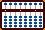Simple Multiplication Ideas Using a Soroban

A soroban is not just a calculation tool. It also helps to develop thinking and analytical skills. And this is a huge advantage. Because of its strong visual reference, a soroban can easily demonstrate that there are often alternative routes one can employ to solve a problem.

For example, what if a student is just beginning to learn the multiplication tables? Once some basic addition and subtraction skills are learned, an abacus can be a powerful tool for learning multiplication and for illustrating some of the basics for how multiplication works. Both examples below assume that a student does not have a full grasp of multiplication tables.

Even though the techniques are quite simple, it might be helpful to have an understanding of a few basic soroban techniques. For more on this please see the addition and subtraction sections at:  Abacus: Mystery of the Bead

Example 1: - Teaching The Seven Times Tables

The following is based on the idea that multiplication is really just a series of additions. For example, if one were to try and teach the 7 times tables using a soroban, this might be one solution.

The idea is simple. In order to come up with the correct answer just continue to add 7's to the abacus.

For those unfamiliar with an abacus it should be said that using complementary number techniques allows students to solve simple addition and subtraction problems in the blink of an eye.

In the following example, I've illustrated the technique by setting both multiplier and multiplicand onto the frame (see rods C and F). This may not be necessary. With a little practice, it's enough to just go ahead and add 7 to rod I in quick succession while mentally keeping track of how many additions you've done.

7 x 1 = 7

Step 1: Set multiplier 1 on rod C  and multiplicand 7 on rod F. Because 7 x 1 = 7, add the product 7 to rod I. ***Note: I've chosen rod I to carry the  unit number in my answer. Any rod on the frame that carries a 'dot' could be substituted.***Fig. 1 ```Step 1 A B C D E F G H I J K L M . . . .   0 0 1 0 0 7 0 0 7 0 0 0 0 ```

7 x 2 = 14

Step 2: Add 1 to the multiplier on rod C to to make it 2.

a) Add 7 to rod I leaving 14 on rods HI.Fig. 2 ```Step 2 A B C D E F G H I J K L M . . . .   0 0 1 0 0 7 0 0 7 0 0 0 0 + 1 Step 2 + 7 Step 2a 0 0 2 0 0 7 0 1 4 0 0 0 0 ```

7 x 3 = 21

Step 3: Add 1 to the multiplier on rod C to to make it 3.

a)  Add 7 to rod I leaving 21 on rods HI.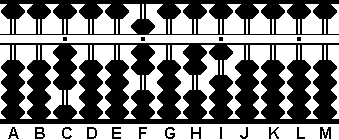Fig. 3 ```Step 3 A B C D E F G H I J K L M . . . .   0 0 2 0 0 7 0 1 4 0 0 0 0 + 1 Step 3 + 7 Step 3a 0 0 3 0 0 7 0 2 1 0 0 0 0```

7 x 4 = 28

Step 4: Add 1 to the multiplier on rod C to to make it 4.

a) Add 7 to rod I leaving 28 on rods HI.Fig. 4 ```Step 4 A B C D E F G H I J K L M . . . .   0 0 3 0 0 7 0 2 1 0 0 0 0 + 1 Step 4 + 7 Step 4a 0 0 4 0 0 7 0 2 8 0 0 0 0 ```

One can continue on to 7 x 12 =  84 or as far as necessary.

**Alternatives**

One could also try variations.

1) For example if a student wanted to find the answer to 7x9=63 an alternative might be double each product 3 times then add 7 one last time ;

7+7=14, 14+14=28, 28+28=56, 56+7=63

Or possibly even the more efficient;

7x10=70 then ==> 70-7=63

2) What if a student is having trouble remembering that 7x8=56. If the student can remember that 8x5=40, use the abacus to quickly add 8 two more times (40+8+8=56), or even more simply add 40+16=56.

There are many variations and combinations one can employ to learn both simple multiplication and how to solve problems.

Example 2:

This next example is another way of solving a multiplication problem. What I like about solving a problem this way is that it teaches students to break down what otherwise might be a complicated problem into more manageable segments. Each segment can be solved and the results added together. This is something I don't remember having learned in school many years ago.

23 x 13 = 299

It's been a long time since I was in school,  but I think I remember that my 10 times tables were quite easy to learn. When multiplying by 10 I learned early on that all I had to do was add a zero to the number being multiplied. With this in mind break down the problem into more manageable segments. Begin by thinking  23 x 10 and place  230 on the abacus.

***Note: Once again, I've chosen rod I to be my unit rod. Any rod on the frame with a 'dot' could be substituted.***

Step 1: Set 230 on rods GHI.Fig. 5 ```Step 1 A B C D E F G H I J K L M . . . .   0 0 0 0 0 0 2 3 0 0 0 0 0 ```

From here we know we have added 23 to the frame 10 times *or* if you prefer we've multiplied 23 x 10 to equal 230. Because the problem is really 23 x 13,  all that's left to do is add 23 three more times times in quick succession to rods HI to end up with the correct answer. A good soroban operator can do this in just a few seconds.

Step 2: Add 23 to rods HI leaving 253 on rods GHI.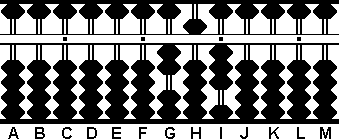Fig. 6 ```Step 2 A B C D E F G H I J K L M . . . .   0 0 0 0 0 0 2 3 0 0 0 0 0 + 2 3 Step 2 0 0 0 0 0 0 2 5 3 0 0 0 0 ```

Step 3: Add 23 to rods HI leaving 276 on rods GHI.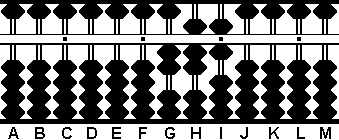Fig. 7 ```Step 3 A B C D E F G H I J K L M . . . .   0 0 0 0 0 0 2 5 3 0 0 0 0 + 2 3 Step 3 0 0 0 0 0 0 2 7 6 0 0 0 0 ```

Step 4: Add 23 to rods HI leaving 299 on rods GHI.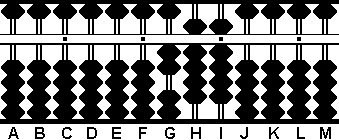Fig. 8 ```Step 4 A B C D E F G H I J K L M . . . .   0 0 0 0 0 0 2 7 6 0 0 0 0 + 2 3 Step 4 0 0 0 0 0 0 2 9 9 0 0 0 ```

And there you have it. 23 x 13 = 299 as shown on rods GHI

The soroban easily demonstrates to students how they might go about solving a problem from different directions. This in turn helps to strengthen an understanding of how numbers work. Not a bad thing at all.